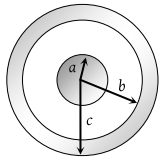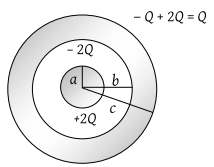A solid conducting sphere of radius a has a net positive charge 2Q. A conducting spherical shell of inner radius b and outer radius c is concentric with the solid sphere and has a net charge –Q. The surface charge density on the inner and outer surfaces of the spherical shell will be(1) $-\frac{2Q}{4\pi {b}^{2}},\frac{Q}{4\pi {c}^{2}}$

(2) $-\frac{Q}{4\pi {b}^{2}},\frac{Q}{4\pi {c}^{2}}$

(3) $0,\frac{Q}{4\pi {c}^{2}}$

(4) None of the above

Concept Videos :-

#22 | Electric Flux
#23 | Gauss Theorem
#24 | Charged Infinite Wire (Gauss Theorem)
#25 | Charged Infinite Sheet (Gauss Theorem)
#26 | Charged Thin Conducting Shell (Gauss Theorem)
#27 | Solid Non Conducting Sphere : Gauss Theorem
#28 | Solid Sphere With Cavity : Gauss Theorem
#29 | Early Model of Atom (Gauss Theorem)
#30 | EFI Inside a Metal (Gauss Theorem)
#31 | Faraday Cages (Gauss Theorem)

Concept Questions :-

Gauss law

(1) Surface charge density (σ)So ${\sigma }_{inner}=\frac{-2Q}{\text{\hspace{0.17em}}4\pi {b}^{2}}$ and ${\sigma }_{Outer}=\frac{Q}{\text{\hspace{0.17em}}4\pi {c}^{2}}$

Difficulty Level:

• 48%
• 20%
• 25%
• 9%